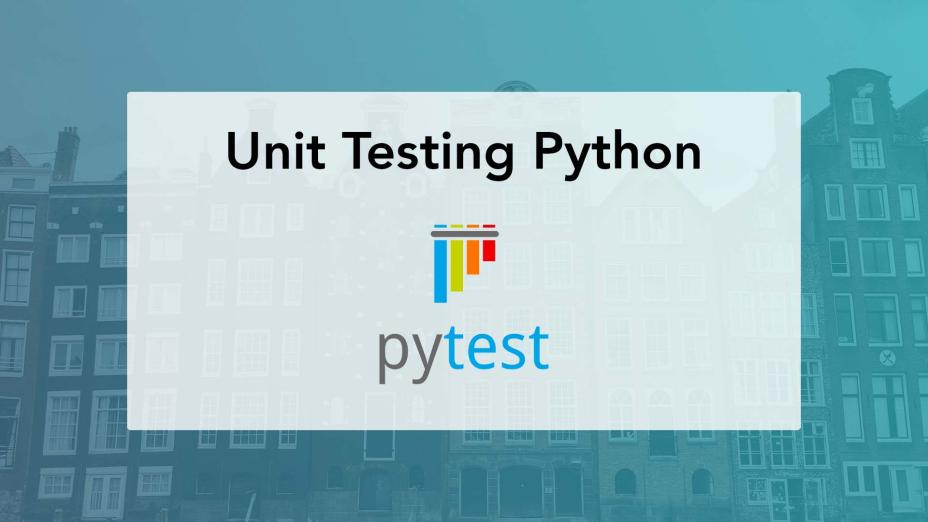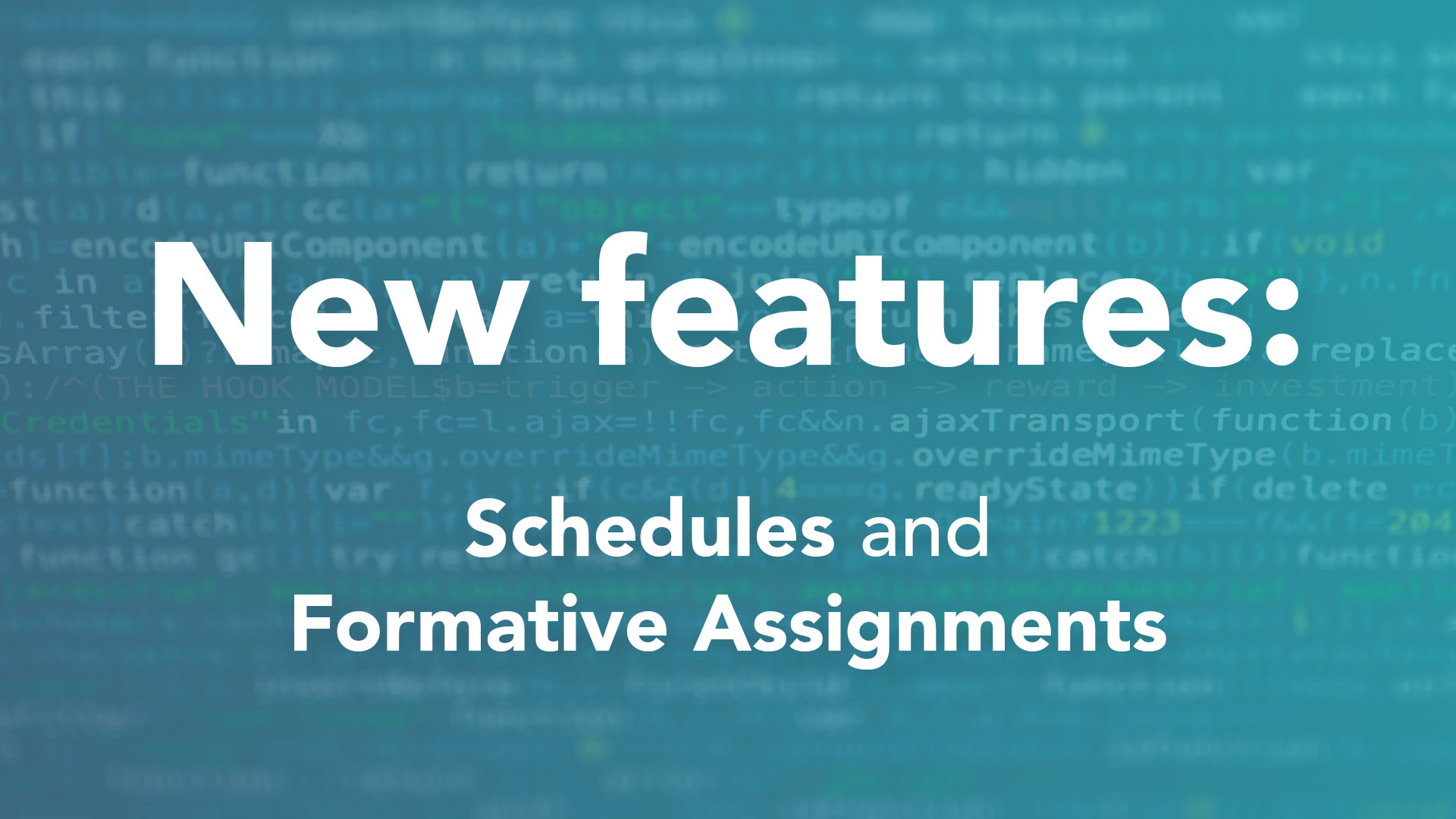Guides
February 8, 2022

# Automatically grading students’ Python assignments using pytest unit tests

### In 30 seconds...

In this blog, we cover:

• Why unit tests are useful for Python courses
• How pytest is used for Python unit testing
• How to create a Python assignment for unit testing
• Setting up a pytest unit test script
• Setting up an AutoTest

Python continues to be one of the most widely used languages on the planet and is one of the most commonly graded languages in CodeGrade too. CodeGrade’s Input/Output (I/O) test step makes grading simple Python scripts a breeze, although it can be limiting when students start developing more complicated programs.

In this guide, I’ll discuss why unit testing could be useful for your python assignment. I will walk you through how you can set up an autograded Python assignment in CodeGrade using the pre-installed pytest unit testing framework.

### Why Unit Test?

As students begin to develop complex programs, it is often accompanied by a segue into the concept of using functions. This encourages students to break down complex programs into manageable steps that each perform one role. In other words, they encapsulate their code using functions.

It is possible to test python functions in CodeGrade using I/O tests by importing the code and calling the functions as you would from any other module. However, by creating a unit test script instead, changing and personalizing your tests becomes much easier. What’s more, many instructors may already have unit test scripts available for their assignments that you can use in CodeGrade to give instant feedback to your students!

CodeGrade has almost all of the industry-standard unit testing tools pre-installed and available in the drop-down menu of the Unit Test step, including pytest. CodeGrade's pytest wrapper, `cg-pytest`, parses the xml produced by running the tests and displays each test separately as its own test step, providing some flexibility in the way you design your unit test setup.

### Pytest for unit testing Python code

Pytest is an open-source python module that enables unit testing of python code in a simple and easy to understand format. The unit testing framework uses simple assertion statements to compare actual outcomes to predicted outcomes. It's designed for writing simple tests but can be scaled for complex functional testing. Read more about pytest and how it works. CodeGrade simply uses pytest under the hood, so all features and documentation of pytest apply to CodeGrade’s implementation too.

### Creating an example Python assignment for unit testing

To demonstrate how we can set up a pytest unit test in CodeGrade, we first need the assignment to be tested. For this situation I have created a simple assignment in which students have been asked to define four basic functions accounting for four mathematical operations: addition, subtraction, multiplication and division. As a small bonus task, we ask the students to handle division by zero by raising an exception. The file they hand in should be called `calculator.py`. Following this prescription we find ourselves with the following code:

-!- CODE language-py -!-def add(x, y):
ans = x + y
return ans

def subtract(x, y):
ans = x + y
return ans

def multiply(x, y):
ans = x * y
return ans

def divide(x, y):

if y ==  0:
raise ValueError("Can not divide by zero!")
ans = x / y
return ans

### Setting up a pytest unit test script

A good unit test makes sure to test not just for the correct cases but for all edge cases and incorrect inputs. In this case, we want to ensure that the student's code does a number of things:

- Returns the correct answer when both numbers are positive integers.

- Returns the correct answer when at least one number is negative.

- Raises a valueError (innate python exception) when dividing by zero.

With these goals in mind we can write out our pytest unit test:

-!- CODE language-py -!-import pytest
import calculator

def  test_subtract():
assert calculator.subtract(5, 2) ==  3
assert calculator.subtract(1, -1) ==  2
assert calculator.subtract(-1, -1) ==  0

def  test_multiply():
assert calculator.multiply(3, 2) ==  6
assert calculator.multiply(1, -1) ==  -1
assert calculator.multiply(-1, -1) ==  1

def  test_divide():
assert calculator.divide(10, 2) ==  5
assert calculator.divide(1, -1) ==  -1
assert calculator.divide(-1, -1) ==  1
assert calculator.divide(5, 2) ==  2.5
with pytest.raises(ValueError):
calculator.divide(10, 0)

### Setting up AutoTest

Now that we have our unit test script prepared we can begin setting up our AutoTest in CodeGrade. We can begin by uploading our unit test script as a fixture. As a general rule, I always recommend including "test_" at the beginning of the file name to make it recognizable. Once uploaded, don't forget to click "submit".

#### Global setup script

Previously, we would have been required to install CodeGrade's pytest wrapper `cg-pytest` in the Global setup but we no longer need to install anything! That's because pytest is installed automatically by CodeGrade's Unit test step, making set up time much quicker and ensuring your students get their AutoTest results as quickly as possible.

#### Per-student setup script

To make our lives easier in the following steps, we can move the unit test script from the \$FIXTURES directory to the \$STUDENT directory where our tests will actually be run. You can do this using a simple bash command in the Per-student setup script:

-!- CODE language-sh -!-mv \$FIXTURES/test_calculator.py \$STUDENT

#### Creating the Unit Test step

Once we've completed the Setup portion of AutoTest we can create a new Level and Category and begin creating our tests. In your new AutoTest category, create a Unit Test step and select the appropriate rubric category. Next, select pytest from the drop-down menu. This should result in a number of additional fields becoming available including "Files to test". In this field, we can enter the file path to our test which, thanks to the command we executed in the previous step, is simply the filename `test_calculator.py` which is in the current (\$STUDENT) directory. Your Unit test step should look like this:

Now, we're all set! All that's left to do is to start our AutoTest and upload a test submission with a correct solution file to see if our tests actually work. Perhaps something interesting to mention here is that this is certainly not the limit to what can be tested in Python using CodeGrade. For instance, CodeGrade also has a number of linters installed by default such as flake8 and pylint which you can use to check if your students' code conforms to industry standards for formatting and code structure. You can also install any number of custom unit test frameworks, linters, or external modules! Want to learn more about autograding Python? Check out our previous webinar which covers grading python step by step…### Samuel Natarajan

Teacher Success Manager
Meet Sam, a music enthusiast! He meticulously ensures CodeGrade clients excel and deeply understand the product, creating an unforgettable experience. He sees CodeGrade's cultural diversity and vibrancy as more than just a workplace.#### New in CodeGrade: Community Library

With the CodeGrade Community Library you can easily import fully automatically graded coding assignments into your own coding course.#### Watch our ChatGPT and Coding Education webinar!

Watch CodeGrade's webinar on using ChatGPT in coding courses to help students work with this new tool and even use it in your grading worklfow yourself!